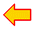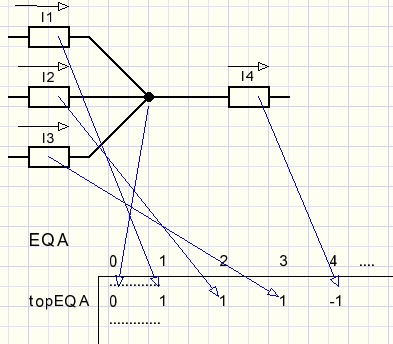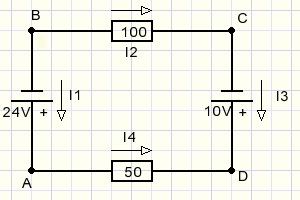DC networks program descriptionterug naar dc-netwerkenback to dc-networkssee Delphi source codesee complete Delphi project

The DC networks project

DC networks is written in the Delphi(7) programming language.
It has the following forms and units:

form1 , unit1

form1 holds:
- a paintbox(1) for network drawing
- paintboxes showing components (wire, resistor, voltage source, ground contact)
- edit boxes to enter component values
- a label for messages
unit1 holds:
- procedures for program control
- event handlers
- paint procedures for the components
Trial painting is done in a bitmap (map2).
Final painting is done in bitmap map1.
While painting in map2 (by mousemovement) map1 is used to erase map2.
map2 is displayed in paintbox1.
Please refer to my other articles where drawing techniques are explained.

network_unit

This unit contains the data formats and procedures to define a network
and perform the calculation of currents and voltages.

resultform,result_unit

They hold a paintbox and procedures to display currents and voltages.

I/O unit

Holds the open and save procedures to write and read networks from disc.

debugform,debug_unit

A paintbox and procedures to display intermediate data (circuits and matrices).
Also flags and a procedure to pause program execution.
Debugging needs code modification at design time.
It cannot be switched on by the user.

This description is limited to the network_unit

The network_unit

Data formats

```const maxelement = 30;
maxcontact = 60;
maxEC      = maxElement + maxContact;

type TElementType = (etNone,etDelete,etWire,etResistor,etVoltage);//the components
TS6 = string;
TElement = record
elType  : TElementtype;
con1    : byte;         //nr of interconnection
con2    : byte;         //..
value   : double;       //Ohm, voltage
vtext   : TS6;          //as entered in edit box
end;
TContact = record
inUse : boolean;
x,y : smallInt;        //coordinate on screen
end;
var element : array[1..maxelement] of Telement;
elCount : byte;                    //number of elements in use
contact : array[1..maxcontact] of TContact;
groundContact : byte;              //number of ground contact
```
In an element current is supposed to flow from contact 1 to contact 2.
(So a negative current flows from 2 to 1).
A new element is entered in the elements[ ] array at the top.
If an element is deleted, the elements positioned above are shifted down.
Variable elCount always points to the top of the elements list.

The contacts behave differently.
An entry in the contact[ ] array holds the boolean variable inUse indicating
that this contact is in use and positioned at (x,y).
If an element is deleted, it"s contacts may still be in use by other elements.

The groundcontact variable equals 0 if no ground is connected.

The registerElement procedure takes care of new elements or deletion of old elements:
```function registerElement(var ep : TElement; x1,y1,x2,y2 : smallInt) : byte;
```
the result is:
0 : element deleted
1 : element replaced
2 : registered OK
3 : max elements reached
4 : fake delete //delete symbol drawn but not matching existing element
To continue I assume that the dcnetworks pages are read where the Kirchhoff laws are explained.

Equation system to solve the currents

```var EQA     : array[0..maxelement,1..maxEC] of double;//equation array
topEQA  : byte;                                   //number of equations
```
A contact results in an equation such as I1 + I2 + I3 - I4 = 0
The I1 value is entered in EQA[1, topEQA] := 1
The I4 value is entered in EQU[4, topEQA] := -1
The 0 value is entered in EQA[0, topEQA] := 0Which elements are connected to a contact?
```var ECV : array[1..maxcontact] of dword;  //element-contact-vector
.....
procedure makeEQA;    //make equation array
var i,j,n,nr : byte;
mf : boolean;     //modify flag
......
begin  //make ECV : bit set for element connected to contact
for j := 1 to maxcontact do ECV[j] := 0;
for i := 1 to elCount do
with element[i] do
begin
end;
```
If element 10 is connected to contact 5 then ECV has bit 10 set.
ECV is used in procedure GetNextFreeElement, see later.

```// Kirchhoff currents

for j := 1 to maxContact do
begin
nr := topEQA + 1;
mf := false;
for i := 1 to elCount do      //Kirchhoff current
with element[i] do
begin
if j = con1 then begin
EQA[i,nr] := 1;
mf := true;
end;
if j = con2 then begin
EQA[i,nr] := -1;
mf := true;
end;
end;//for i
if mf then topEQA := nr;
end;//for j
```
Well, this was the easy part.
Next comes the implementation of Kirchhoff's current law.The path A...B...C...D...A is called a circuit.
Starting from A we use Ohm's law (E = I.R) to write the equation
-24 -100I2 +10 +50I4 = 0 ...........or
-100I2 + 50I4 = 14
To solve this system we need also the previously found equations
I2 - I3 = 0
I3 + I4 = 0
So, the EQA matrix looks like:So, per element we have to find a circuit to apply Kirchhoff's voltage law.
Below I describe the method to find the shortest circuit for each element.

Finding circuits

Data structures:
```type Tcircuit = record
ctIn,ctOut : byte;       //entry contact
el : byte;               //element
mult : shortInt;         //1, -1  multiplier
end;

var circuit : array[1..maxContact] of Tcircuit;
ccLength: byte;
ECV     : array[1..maxcontact] of dword;  //element-contact-vector
CEF     : array[1..maxcontact] of boolean;//contact enable flag
EEF     : array[1..maxelement] of boolean;//element enable flag
CCOK    : boolean;                        //circuit OK
```
The boolean array CEF[ ] enables contacts.
A false flag prevents that the contact is selected (again).
Array EEF[ ] enables elements and prevent re-election of an element already part of a circuit.
The circuit array circuit[ ] holds the elements that make a circuit.
Variable mult (sign multiplier) equals 1 if ctIn = con1 and ctOut = con2.
mult = -1 if ctIn = con2 and ctOut = con1, when current flows reverse.
Remember that current is assumed to flow from element[ ].con1 to element[ ].con2 contacts.

One of the arrays CEF, EEF seems superfluous.
When searching for a circuit it is sufficient not to select contacts
that are already part of the circuit.
However, as the last contact in a circuit the starting contact must be choosen.
This contact should not be excluded. Elements may never appear twice in a circuit.
So I record both elements and contacts.

Following procedure is called when the analyse button is pressed:
```procedure makeEQA;   //make equation array
var i,j,n,nr : byte;
mlt  : shortInt; //sign multiplier for current direction
begin
......
// Kirchhoff voltages

for i := 1 to elCount do     //for all elements
begin
ccLength := 0;             //reset circuit length
if findCircuit(i) then     //if circuit found
begin
inc(topEQA);
for j := 1 to ccLength do
begin
with circuit[j] do     //translate circuit data to equation
begin
n := el;
mlt := mult;
end;
with element[n] do
begin
case eltype of
etResistor : EQA[n,topEQA] := mlt*value;
etVoltage  : EQA[0,topEQA] := EQA[0,topEQA] + mlt*value;
end;//case
end;
end;//for j
end;//if find..
end;//for i
end;
```
MakeEQA calls findcircuit to.....(indeed).
This function exits true if a circuit is found.
Parameter fel is the first element of the circuit.
Below I describe what is happening, see the listing for details.
```function findcircuit(fel : byte)  : boolean;
//...a circuit made in array node[ ] is
//only stored in array circuit[ ] when it is shorter
var i : byte;
node : array[1..maxElement] of TCircuit;
nn,nel : byte; //node nr
label nextNode,nextMove;
begin
.....
//...preset CEF, EEF,Nodes
....
//...set first node (element, contacts)
.....
nextnode:
.....
//...increment node number nn
//   set ctIn to ctOut of previous node
.....
nextmove:
.....
//...if free element found
//      set element, contacts in node[ ]
//      if round trip
//         set result true
//         if shorter replace circuit[ ] by node[ ]
//      no round trip...goto nextNode
//.....
//...(move back to previous node)
//      re-enable element, contacts in EEF,ECF
//      remove node
//      goto nextMove
```
After finding a circuit, the last element is removed and
control is returned to the previous node
to find more (maybe shorter) circuits.

To find a free element:
```function GetNextFreeElement(var el:byte; ct:byte) : boolean;
//get next free element connected to contact ct
//drop contact and element enables
var n,conOut : byte;
begin
result := false;
n := el;
while (result = false) and (n < elCount) do
begin
inc(n);
result := EEF[n] and (((1 shl n) and ECV[ct]) > 0);
if result then
with element[n] do
begin
if con1 = ct then conOut := con2 else conOut := con1;
result := CEF[conOut];
end;
end;
if result then
with element[n] do
begin
EEF[n] := false;   //prevent using them again
CEF[con1] := false;
CEF[con2] := false;
el := n;
end;
end;
```
This concludes the construction of EQA, the equations array.

Solving the equations in EQA

This is done by Gauss-Jordan elimination.
A description is found [ here ].

Below a summary of the work:
```procedure solveEQA;
var i,j,k,n,c1,c2 : byte;
HV,M,D : double;
nochange : boolean;
begin
...
//.....sweep columns down to zero
...
//.....sweep columns to zero from bottom to top
...
//At this point all entries in EQA[..,..] are zero except for EQA[i,i]
...
//.....divide rows to make diagonal all  = 1
...
//.....check for non zero values on diagonal (error check)
...
//.....if ground connected
//        make voltage list starting at groundcontact
```
This concludes the DC-networks project description.
Please refer to the source code for details.OneStat